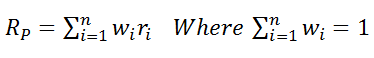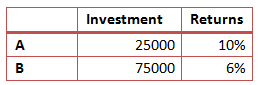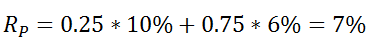How to Calculate Portfolio Returns

We have learned about how to calculate the returns on single assets. However, portfolio managers will have many assets in their portfolios in different proportions. The portfolio manager will have to therefore calculate the returns on the entire portfolio of assets. The returns on the portfolio are calculated as the weighted average of the returns on all the assets held in the portfolio.

The formula for portfolio returns is presented below:w represents the weights of each asset, and r represents the returns on the assets. For example, if an asset constitutes 25% of the portfolio, its weight will be 0.25. Note that sum of all the asset weights will be equal to 1, as it will represent 100% of the investment. The returns here are single period returns with same periods for each asset’s returns.

Let’s take an example of a two asset portfolio to understand how portfolio returns are calculated. Let’s say that our portfolio comprises of two assets A and B and has the following details.The table presents the amount invested in each asset and the returns from each asset. The total amount invested is \$100,000. We can calculate the weights for each asset as follows:

wA = 25000/100000 = 0.25

wB = 75000/100000 = 0.75

We can now calculate the portfolio returns as follows:The same calculation can be extended for multiple assets.

In the later articles we will also learn how to calculate the risk of the portfolio.